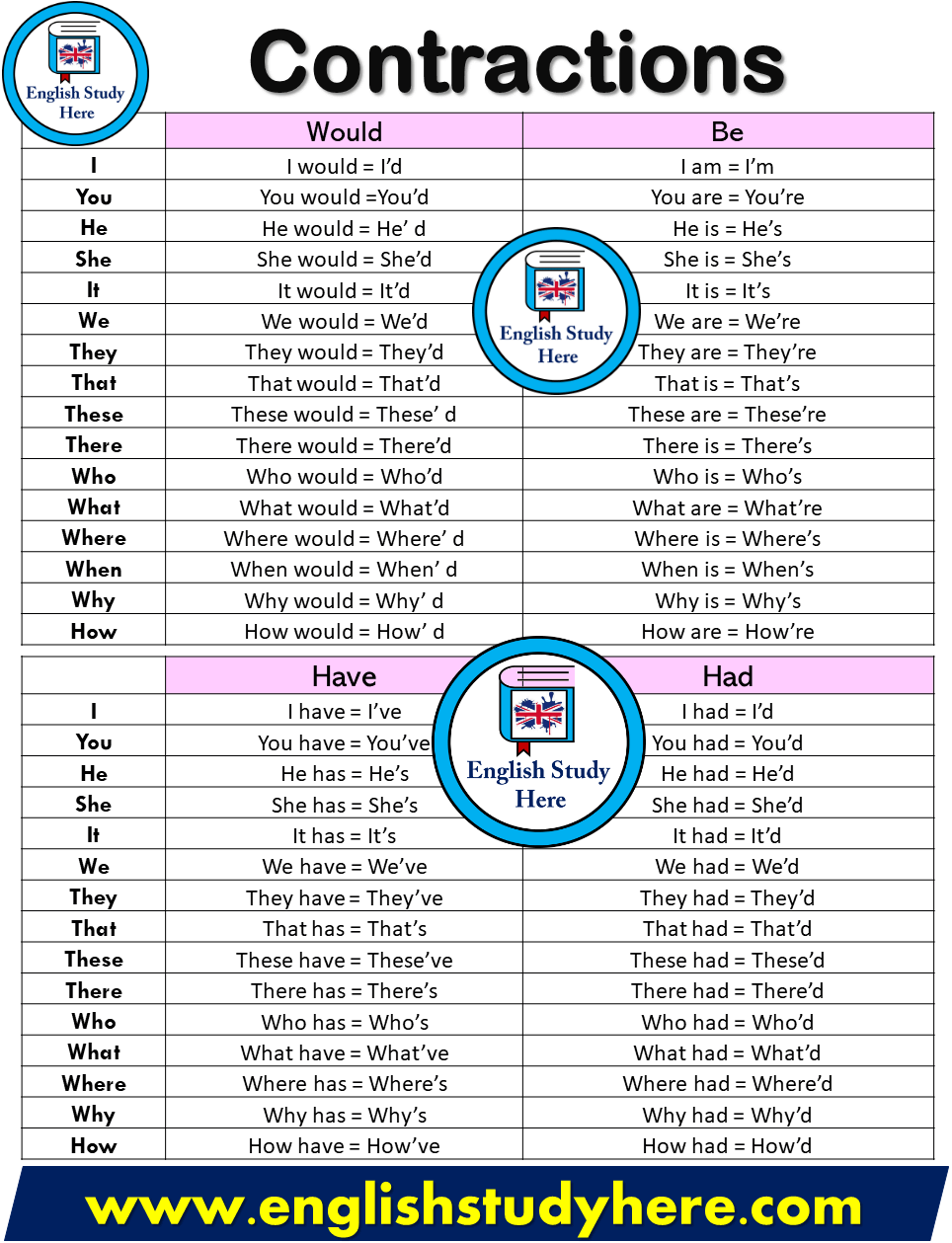# Contractions List

Contractions List in english;Would Be I I would = I’d I am = I’m You You would =You’d You are = You’re He He would = He’ d He is = He’s She She would = She’d She is = She’s It It would = It’d It is = It’s We We would = We’d We are = We’re They They would = They’d They are = They’re That That would = That’d That is = That’s These These would = These’ d These are = These’re There There would = There’d There is = There’s Who Who would = Who’d Who is = Who’s What What would = What’d What are = What’re Where Where would = Where’ d Where is = Where’s When When would = When’ d When is = When’s Why Why would = Why’ d Why is = Why’s How How would = How’ d How are = How’re